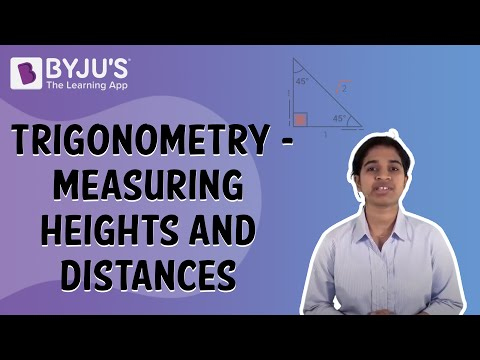# Some Applications Of Trigonometry Class 10 Notes- Chapter 9

## Heights and Distances

### Horizontal Level and Line of Sight

Line of sight is the line drawn from the eye of the observer to the point on the object viewed by the observer.

Horizontal level is the horizontal line through the eye of the observer.

To know more about Height and Distance, visit here.

### Angle of elevation

The angle of elevation is relevant for objects above horizontal level.
It is the angle formed by the line of sight with the horizontal level.

To know more about Angle of elevation, visit here.

### Angle of depression

The angle of depression is relevant for objects below horizontal level.
It is the angle formed by the line of sight with the horizontal level.

To know more about Angle of depression, visit here.

### Calculating Heights and Distances

To, calculate heights and distances, we can make use of trigonometric ratios.

Step 1: Draw a line diagram corresponding to the problem.

Step 2: Mark all known heights, distances and angles and denote unknown lengths by variables.

Step 3: Use the values of various trigonometric ratios of the angles to obtain the unknown lengths from the known lengths.

To get solved problems on Heights and Distances, visit here.

#### For More Information On Trigonometry – Measuring Heights And Distances, Watch The Below Video:## Be More Curious

### Measuring the distances of Celestial bodies with the help of trigonometry

Large distances can be measured by the parallax method. The parallax angle is half the angle between two line of sights when an object is viewed from two different positions. Knowing the parallax angle and the distance between the two positions, large distances can be measured.

Test your knowledge on Some Application Of Trigonometry For Class 10

#### 1 Comment

1. Jessica

This is really very helpful me bcz I am getting previous problems also😊😊😊😔